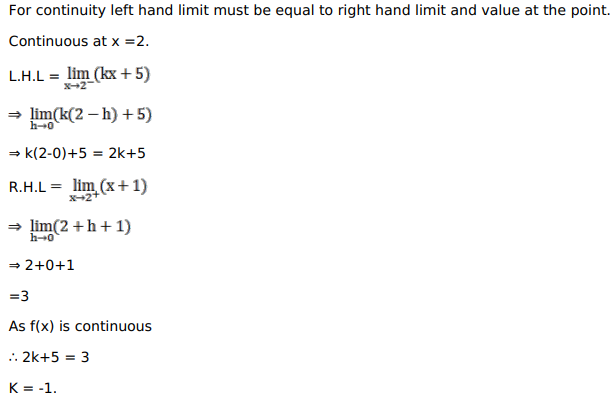# Solve this following

Question:

Mark $(\sqrt{)}$ against the correct answer in the following:

If $f(x)=\left\{\begin{array}{c}\mathrm{kx}+5, \text { when } \mathrm{x} \leq 2 \\ \mathrm{x}+1, \text { when } \mathrm{x}>2\end{array}\right.$ is continuous at $\mathrm{x}=2$ then $\mathrm{k}=?$

A. 2

B. $-2$

C. 3

D. $-3$

Solution: